﻿ 湛江港附近海域的海上垃圾的聚集点研究

# 湛江港附近海域的海上垃圾的聚集点研究Study on the Accumulation of Marine Garbage in the Sea Area near Zhanjiang Port

Abstract: Marine environmental governance is a direction worthy of in-depth study. With the rapid devel-opment of social economy, there are more and more industrial wastes and domestic wastes. As garbage seeps from coastal areas to the deep sea, the marine ecological environment has been damaged. In order to deal with these marine wastes, the relevant departments in our country need to invest a large amount of cost to deal with them. How to clean up and recycle marine wastes effi-ciently has become an urgent problem to be solved. In this paper, taking the East Island as an ex-ample, the prediction of waste accumulation points in Zhanjiang Bay is carried out: according to the transport effect of coastal current on garbage and the calculation of flow intersection point, the garbage collection point model is established, and the garbage distribution point is obtained through simulation calculation; then, a weight model based on the distance between current and coast is established to give reasonable weight to each garbage collection point; and then, the weighted K-means clustering analysis is used to estimate the garbage concentration center. The results can provide the relevant departments with the location of the garbage collection center, facilitate the garbage disposal on the sea surface, and provide a good reference for the local marine environmental protection.

1. 引言

2. 集聚点模型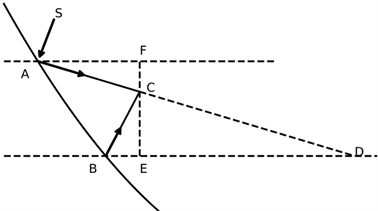Figure 1. Schematic diagram of flow intersection

$\frac{CE}{BE}=\mathrm{tan}{\theta }_{2}=\frac{{y}_{1}-{y}_{2}}{{x}_{3}-{x}_{2}},\text{ }\frac{CF}{AF}=\mathrm{tan}{\theta }_{1}=\frac{{y}_{3}-{y}_{1}}{{x}_{3}-{x}_{1}},\text{ }\frac{CE}{DE}=\mathrm{tan}{\theta }_{1}=\frac{{y}_{3}-{y}_{2}}{{x}_{3}-{x}_{D}},$ (1)

$\frac{{y}_{3}-{y}_{1}}{{x}_{3}-{x}_{1}}=\frac{\mathrm{tan}{\theta }_{2}\left({x}_{3}-{x}_{2}\right)+{y}_{2}-{y}_{1}}{{x}_{3}-{x}_{1}}=\mathrm{tan}{\theta }_{1},$ (2)

$\mathrm{tan}{\theta }_{2}\left({x}_{3}-{x}_{2}\right)+{y}_{2}-{y}_{1}=\mathrm{tan}{\theta }_{1}\left({x}_{3}-{x}_{1}\right)$,于是得到如下垃圾集聚点C的计算式：

$\left\{\begin{array}{l}{x}_{3}=\frac{{y}_{1}-{y}_{2}-\mathrm{tan}{\theta }_{1}{x}_{1}+\mathrm{tan}{\theta }_{2}{x}_{2}}{\mathrm{tan}{\theta }_{2}-\mathrm{tan}{\theta }_{1}}\\ {y}_{3}=\mathrm{tan}{\theta }_{2}\left({x}_{3}-{x}_{2}\right)+{y}_{2}\end{array}$ (3)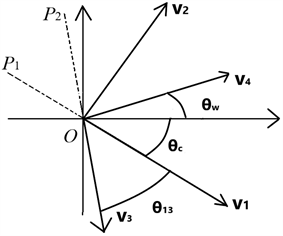Figure 2. Reflection diagram

$\left\{\begin{array}{l}{\theta }_{13}=\mathrm{arccos}\left(\frac{{v}_{3}\cdot {v}_{1}}{|{v}_{3}||{v}_{1}|}\right)\\ {\theta }_{w}=|{\theta }_{13}|+{\theta }_{c}\end{array}$ (4)

3. 水速下降与集聚点权重模型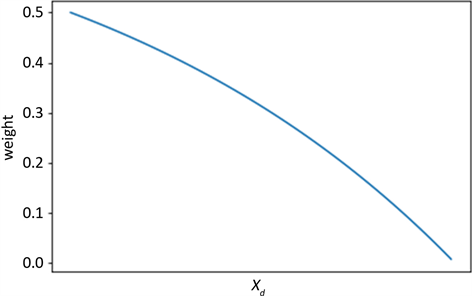Figure 3. Variation curve of current weight with coastal distance

4. 集聚点中心预测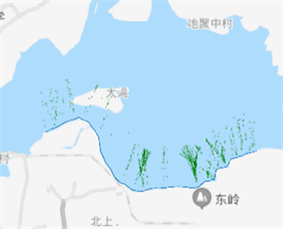Figure 4. Distribution of garbage accumulation sites in the north of Donghai Island, Zhanjiang Bay

K-Means算法描述如下：

1. 指定聚类中心的数量k，随机初始化k个聚类中心；

2. 计算每个点到各个中心的距离，距离最小的中心记为与该点同类；

3. 将每一类的点的加权平均更新为每一类的中心；

4. 重复2~3至每一类的中心不再发生变化。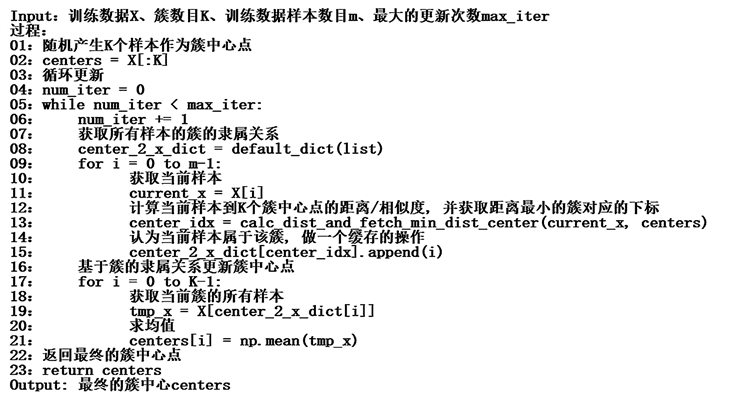Figure 5. Pseudo code of K-Means algorithm

5. 东海岛北海岸垃圾预测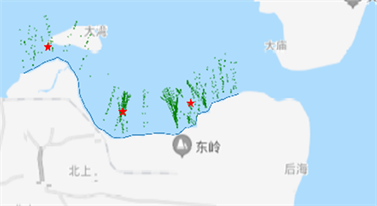Figure 6. Garbage collection point and its center on the North Bank of Donghai Island (northwest wind)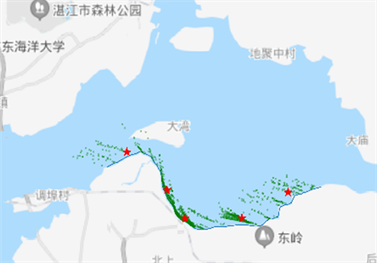Figure 7. Garbage collection point and its center on the North Bank of Donghai Island (northeast wind)

6. 总结

 冯国艳. 湛江湾海岸带综合管理优化研究[D]: [硕士学位论文]. 湛江: 广东海洋大学, 2019.

 杨越, 陈玲, 薛澜. 寻找全球问题的中国方案: 海洋塑料垃圾及微塑料污染治理体系的问题与对策[J]. 中国人口•资源与环境, 2020(10): 45-52.

 刘必劲. 近岸Duck Mouth型海漂垃圾收集装备结构型式及水动力研究[D]: [博士学位论文]. 大连: 大连理工大学, 2019.

 林海卓, 杨哲, 辛博, 占海明. 基于阻尼振荡动态模拟环太平洋垃圾带的成因[J]. 科技传播, 2010(16): 108-109.

 周艺. 基于中心聚集参数的改进K-means协同过滤推荐算法[D]: [硕士学位论文]. 石家庄: 河北经贸大学, 2020.

 林竹明. 海上养殖区海漂垃圾收集方法研究综述[J]. 山西建筑, 2016, 42(27): 182-184.

 赵芸莹. 针对海洋固体废物处理与回收的研究[J]. 小作家选刊(教学交流), 2018(1): 274.

 杨光. 如何加强海洋垃圾污染防治工作[J]. 资源节约与环保, 2020(3): 16.

 马利霞. 中国沿海地区陆地塑料垃圾入海量估算[D]: [硕士学位论文]. 天津: 天津师范大学, 2019.

 许阳. 中国海洋环境治理政策的概览、变迁及演进趋势——基于1982-2015年161项政策文本的实证研究[J]. 中国人口•资源与环境, 2018, 28(1): 165-176.

Top